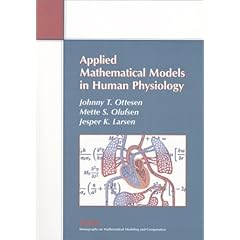Applied Mathematical Models in Human Physiology: This book introduces mathematicians to real applications from physiology. Using mathematics to analyze physiological systems, the authors focus on models reflecting current research in cardiovascular and pulmonary physiology. In particular, they present models describing blood flow in the heart and the cardiovascular system, as well as the transport of oxygen and carbon dioxide through the respiratory system and a model for baroreceptor regulation.

Applied Mathematical Models in Human Physiology is the only book available that analyzes up-to-date models of the physiological system at several levels of detail. Some are simple "real-time" models that can be directly used in larger systems, while others are more detailed "reference" models that show the underlying physiological mechanisms and provide parameters for and validation of simpler models. The book also covers two-dimensional modeling of the fluid dynamics in the heart and its ability to pump, and includes a discussion of modeling wave-propagation throughout the systemic arteries.

The models presented can be used as case studies in courses on mathematical modeling or as an inspiration for the study and development of physiological models. The first two chapters of the book provide an excellent introduction to the physiologic concepts necessary for understanding the modeling assumptions and methodologies. The remaining chapters describe six different models of the cardiovascular and pulmonary system. Each model is introduced by a case study and can be studied individually.